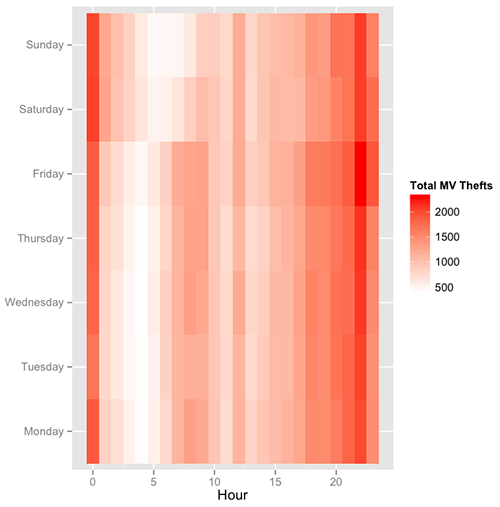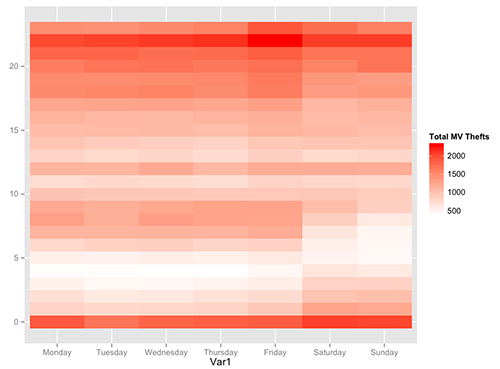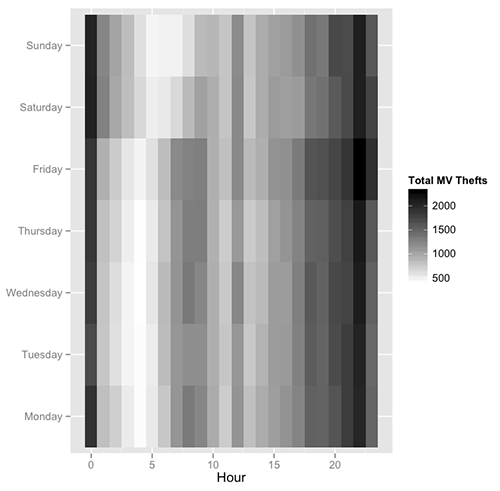15.071 | Spring 2017 | Graduate

# The Analytics Edge

7.3 The Analytical Policeman: Visualization for Law and Order

## 7.3 The Analytical Policeman: Visualization for Law and Order

### Quick Question

In this quick question, we’ll ask you questions about the following plots. Plot (1) is the heat map we generated at the end of Video 4. Plot (2) and Plot (3) were generated by changing argument values of the command used to generate Plot (1).

Plot (1)Plot (2)Plot (3)Which argument(s) did we change to get Plot (2)? Select all that apply.

x  check
y  check
fill  close
name  close
low  close
high  close

Explanation To get Plot (2), we changed the arguments "x" and "y" (we flipped them). Plot (2) can be generated with the following code: ggplot(DayHourCounts, aes(x = Var1, y = Hour)) + geom\_tile(aes(fill=Freq)) + scale\_fill\_gradient(name="Total MV Thefts", low="white", high="red") + theme(axis.title.y=element\_blank())

Which argument(s) did we change to get Plot (3)? Select all that apply.

x  close
y  close
fill  close
name  close
low  close
high  check

Explanation To get Plot (3), we changed the argument "high" to "black". Plot (3) can be generated with the following code: ggplot(DayHourCounts, aes(x = Hour, y = Var1)) + geom\_tile(aes(fill=Freq)) + scale\_fill\_gradient(name="Total MV Thefts", low="white", high="black") + theme(axis.title.y=element\_blank())

## Course Info

Spring 2017
##### Learning Resource Types
Lecture Videos
Lecture Notes
Problem Sets with Solutions